# Density-Based Clustering - DBSCAN, OPTICS & DENCLUE

## Density-Based Clustering

Density-Based Clustering method is one of the clustering methods based on density (local cluster criterion), such as density-connected points.

The basic ideas of density-based clustering involve a number of new definitions. We intuitively present these definitions and then follow up with an example.

The neighborhood within a radius ε of a given object is called the ε-neighborhood of the object.

If the ε-neighborhood of an object contains at least a minimum number, MinPts, of objects, then the object is called a core object.

### Density-reachable:

• A point p is density-reachable from a point q wrt. Eps, MinPts if there is a chain of points p1, …, pn, p1 = q, pn = p such that pi+1 is directly density-reachable from pi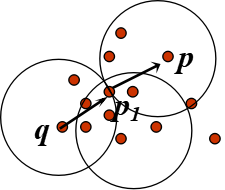### Density-connected

• A point p is density-connected to a point q wrt. Eps, MinPts if there is a point o such that both, p and q are density-reachable from o wrt. Eps and MinPts.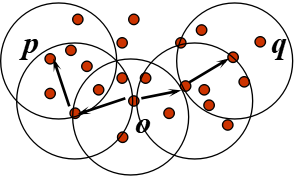## Working Of Density-Based Clustering

Given a set of objects, D' we say that an object p is directly density-reachable from object q if p is within the ε-neighborhood of q, and q is a core object.

An object p is density-reachable from object q with respect to ε and MinPts in a set of objects, D' if there is a chain of objects p1,.,.,.pn, where p1 = q and pn = p such that pi+1 is directly density-reachable from pi with respect to e and MinPts, for 1/n, pi € D.

An object p is density-connected to object q with respect to ε and MinPts in a set of objects, D', if there is an object o, belongs D such that both p and q are density-reachable from o with respect to ε and MinPts.

## Density-Based Clustering - Background

Two parameters:
• Eps: Maximum radius of the neighborhood.
• MinPts: Minimum number of points in an Eps-neighbourhood of that point.
NEps(p): {q belongs to D | dist(p,q) <= Eps}
Directly density-reachable: A point p is directly density-reachable from a point q wrt. Eps, MinPts if
• p belongs to NEps(q)
• core point condition:|NEps (q)| >= MinPts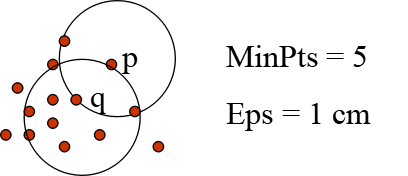## Major features:

It is used to discover clusters of arbitrary shape.

It is also used to handle noise in the data clusters.

It is a one scan method.

It needs density parameters as a termination condition.

## Density-Based Methods

DBSCAN: Ester, et al. (KDD’96)

OPTICS: Ankerst, et al (SIGMOD’99).

DENCLUE: Hinneburg & D. Keim  (KDD’98)

CLIQUE: Agrawal, et al. (SIGMOD’98)

## DBSCAN(Density-Based Spatial Clustering of Applications with Noise)

It relies on a density-based notion of cluster:  A cluster is defined as a maximal set of density-connected points.

It discovers clusters of arbitrary shape in spatial databases with noise.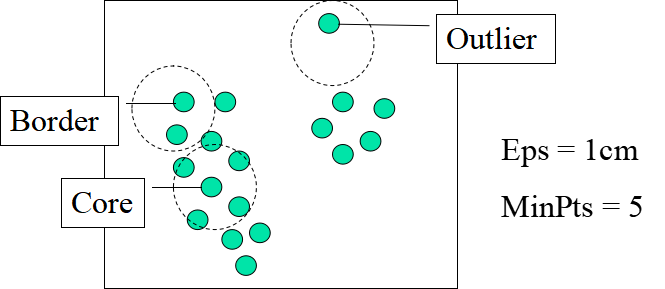## DBSCAN Algorithm

Arbitrary select a point p.

Retrieve all points density-reachable from p wrt Eps and MinPts.

If p is a core point, a cluster is formed.

If p is a border point, no points are density-reachable from p and DBSCAN visits the next point of the database.

Continue the process until all of the points have been processed.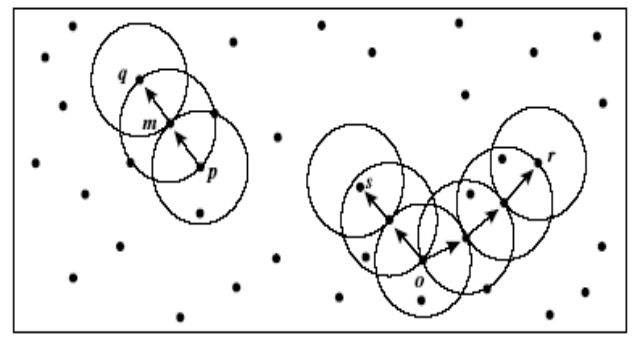say, let MinPts = 3.

Of the labeled points, m, p, o, and r are core objects because each is in an ε-neighborhood containing at least three points.

q is directly density-reachable from m. m is directly density-reachable from p and vice versa.

q is (indirectly) density-reachable from p  because q is directly density-reachable from m and m is directly density-reachable from p.

However, p is not density-reachable from q  because q is not a core object.

Similarly, r and s are density-reachable from o, and o is density-reachable from o, and o is density-reachable from R.

## OPTICS - A Cluster-Ordering Method

OPTICS: Ordering Points To Identify the Clustering Structure.
• It produces a special order of the database with respect to its density-based clustering structure.
• This cluster-ordering contains info equivalent to the density-based clusterings corresponding to a broad range of parameter settings.
• It is good for both automatic and interactive cluster analysis, including finding an intrinsic clustering structure.
• It can be represented graphically or using visualization techniques.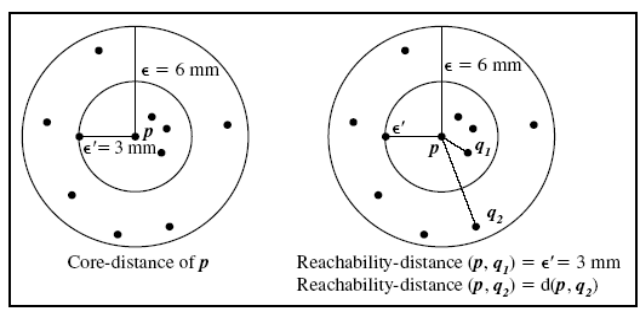Core-distance and reachability-distance: The figure illustrates the concepts of core-distance and reachability-distance.

Suppose that e=6 mm and MinPts=5.
The core distance of p is the distance, e0, between p and the fourth closest data object.

The reachability-distance of q1 with respect to p is the core-distance of p (i.e., e0 =3 mm) because this is greater than the Euclidean distance from p to q1.

The reachability distance of q2 with respect to p is the Euclidean distance from p to q2 because this is greater than the core-distance of p.

## DENCLUE - Using Density Functions

DENsity-based CLUstEring by Hinneburg & Keim  (KDD’98)

Major Features
• It ha got a solid mathematical foundation.
• It is definitely good for data sets with large amounts of noise.
• It allows a compact mathematical description of arbitrarily shaped clusters in high-dimensional data sets.
• It is significantly faster than the existing algorithm (faster than DBSCAN by a factor of up to 45).
• But it needs a large number of parameters.

## DENCLUE - Technical Essence

It uses grid cells but only keeps information about grid cells that do actually contain data points and manages these cells in a tree-based access structure.

Influence function: This describes the impact of a data point within its neighborhood.

The Overall density of the data space can be calculated as the sum of the influence function of all data points.

The Clusters can be determined mathematically by identifying density attractors.

The Density attractors are local maxima of the overall density function.

## Summary

Density-Based Clustering -> It is one of the clustering methods based on density (local cluster criterion), such as density-connected points.

Subscribe us for more content on Data.